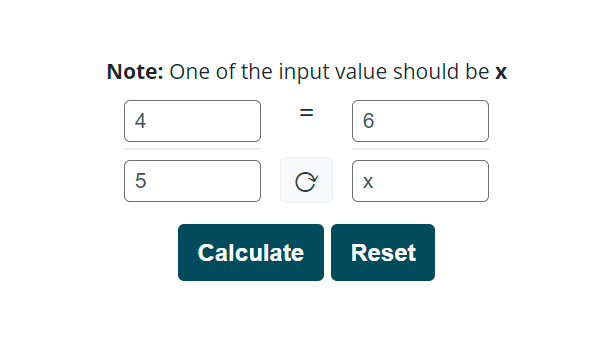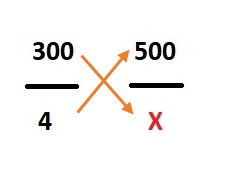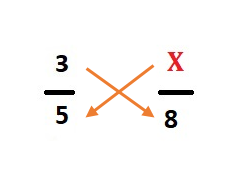# Proportion Calculator

Enter the 3 input values, the 4rth input should be unknown (x) and hit the calculate button to find the proportion between two fractions using this proportion calculator/solver.

=## Proportion Solver

Solve the value of an unknown variable using this proportion calculator. It is an online tool that assists you in solving proportions without much effort. In fact, it makes the process of solving proportions quite effortless. You only have to put the values and a variable X in the given boxes to get the value of X.

Do you know what a proportion is? How do you calculate an unknown variable in a proportion manually? Is there any difference between proportion and fraction?

Here, in this content, we will answer all those questions. Furthermore, we will discuss proportion definition, how to solve proportions with examples, and much more. So, stay tuned to this space.

## How does the proportion calculator work?

In this section, we will explain how to find proportions using a calculator?

Manually, we calculate the unknown variable in a proportion by using cross multiplication method. It will come up in the next section, though. First, let’s see how we can use this cross multiplication calculator to find a proportion.

As you know, in proportion, we already have three values with us and we try to find the 4th one. That missing value is denoted by X in most cases. To use this calculator, follow the steps below.

• Enter the numerator of 1st fraction in the first input box on the left side.
• Enter the denominator of 1st fraction in the second input box on the left side.
• Enter the numerator of 2nd fraction in the first input box on the right side.
• Enter the denominator of 2nd fraction in the second input box on the right side.
• Out of these four values, one must be the variable So, enter X in an input box mentioned above according to the position of missing value in the fraction.

Suppose, we have two fractions:

4/x and 3/2

Put the values as shown in the image below.• Hit the Calculate button to get the value of
• Use the Reset button to enter new values.

Our ratio calculator shows the missing value in decimal. If you want this value in percentage, you can use our percentage calculator. Moreover, the missing value of proportion can also be stated in fraction form. Use our fraction calculator if you need to calculate fractions.

## What is the proportion?

A proportion represents the relationship between two quantities. It consists of two ratios of two fractions joined by an equal sign “=”. A proportion can be written as:

2 : 5 = 7 : 4 or

2/5 = 7/4

Wikipedia defines proportion as,

In mathematics, two varying quantities are said to be in a relation of proportionality, if they are multiplicatively connected to a constant; that is when either their ratio or their product yields a constant. The value of this constant is called the coefficient of proportionality or proportionality constant.

There are mainly three types of proportions.

Direct proportion: If the quantity of one variable increases, the quantity of another variable also increases, and vice versa. It is known as a direct proportion.

Inverse proportion: If the quantity of one variable increases, the quantity of other variable decreases and vice versa. It is known as the inverse proportion.

Compound proportion: It is a type of proportion which deals with two or more quantities at a time. I.e. 2 : 3 : 4 = 3 : 7 : 1

## How to solve a proportion?

Students have to solve proportions by hand in their exams and classrooms. This tool can be very helpful to quickly complete the assignments and homework. Moreover, it can be used to learn the calculations of proportion. Here, we will illustrate how to calculate proportion by yourself.

### Cross multiplication method

To calculate a missing value or unknown variable in a proportion, follow the below steps:

• Write the given values in fraction form. Use any variable to represent the unknown value.
• Join both fractions using an equal sign.
• Multiply both fractions diagonally. I.e. multiply the numerator of the first fraction with the denominator of the second fraction and multiply the numerator of the second fraction with the denominator of the first fraction.
• Write both numbers after multiplication and put an equal sign between them.
• Find the value of the variable by isolating it on either side of the equation.

### Example – Direct proportion

A train from Seoul to Busan travels 300 km distance in 4 hours. How much time will it take to reach a distance of 500 km?

Solution:

Step 1: Write the given values in fraction form. Use any variable to represent the unknown value.

It travels 300 km in 4 hours, the ratio will be: 300 / 4

The ratio for the distance of 500 km will be: 500 / X

Step 2: Join both fractions using an equal sign.

300 / 4 = 500 / X

Or

300 : 4 = 500 : X

Step 3: Multiply both fractions diagonally. I.e. multiply the numerator of the first fraction with the denominator of the second fraction and multiply the numerator of the second fraction with the denominator of the first fraction.Step 4: Write both numbers after multiplication and put an equal sign between them.

300 X = 500 × 4

Step 5: Find the value of the variable by isolating it on either side of the equation.

X = 2000 / 300

X = 6.6 hours approx.

So, the train will take approximately 6 and a half hours to covers a distance of 500 km.

### Example – Inverse proportion

In a toy manufacturing factory, 3 workers make a box of toys in 8 days. The company has hired 2 more workers to increase the production of the unit. There are a total of 5 workers in the factory now. How long will it take to complete that same task by 5 workers?

Solution:

Step 1: Write the given values in fraction form. Use any variable to represent the unknown value.

Ratio of workers before and after = 3 / 5

Ratio of days of completion = X / 8

Step 2: Join both fractions using an equal sign.

3 / 5 = X / 8

Step 3: Multiply both fractions diagonally. I.e. multiply the numerator of the first fraction with the denominator of the second fraction and multiply the numerator of the second fraction with the denominator of the first fraction.Step 4: Write both numbers after multiplication and put an equal sign between them.

5 X = 3 × 8

Step 5: Find the value of the variable by isolating it on either side of the equation.

X = 24 / 5

X = 4.8

So, 5 workers will manufacture the toys in approx. 4.8 days.

## FAQs

### How do you calculate proportion?

Proportion can be calculated by using a cross multiplication method. In the cross multiplication method, we diagonally multiply the numerator and denominator of both fractions and calculate the value of an unknown variable by isolating it on one side of the equation.

For example, we have two fractions as:

2/3 = 4/x

By cross multiplication, we get:

2x = 12 è x = 6

### What are examples of proportions?

The followings are some examples of proportions:

• We use proportions of ingredients for cooking a specific amount of food.
• We compare the prices of various shopping centers by using proportions.
• Builders mix sand and cement to the gravel to make a solution by using the specific proportions.
• Chemists make several chemical formulas and medicines by using proportions of various chemicals and drugs.
• A rope of specific weight and length. The length and weight of the rope are proportional.
• The sizes of the shapes of any objects can be proportional to each other.

### What is the ratio of 4 to 3?

The ratio of 4 to 3 can be written as 4 : 3. It means that the second quantity is 1/3 of the first quantity.

A few equivalent ratios of 4 : 3 are:

 4 : 3 8 : 6 12 : 9 16 : 12 20 : 15 24 : 18 28 : 21 32 : 24 36 : 27 40 : 30 44 : 33 48 : 36 52 : 39 56 : 42 60 : 45 64 : 48 68 : 51 72 : 54 76 : 57 80 : 60 84 : 63 88 : 66 92 : 69 96 : 72 100 : 75

### What is the ratio of 1 to 5?

A ratio of 1 to 5 can be written as 1 : 5. It shows that the quantity in second place is five-time the quantity in the first place. Some equivalent ratios of 1 : 5 are:

 1 : 5 2 : 10 3 : 15 4 : 20 5 : 25 6 : 30 7 : 35 8 : 40 9 : 45 10 : 50 11 : 55 12 : 60 13 : 65 14 : 70 15 : 75 16 : 80 17 : 85 18 : 90 19 : 95 20 : 100 21 : 105 22 : 110 23 : 115 24 : 120 25 : 125